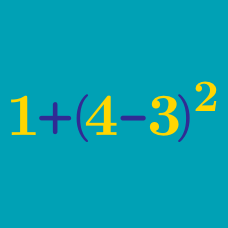Basic Mathematics

Representation on the Real Line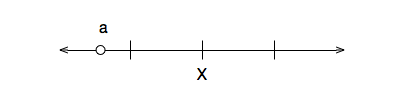In the above real line, if $X = 3$, which of the following is a possible value for $a?$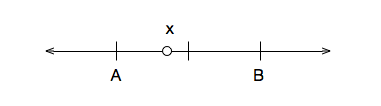In the above real line, if $A = 2$ and $B = 4$, which of the following is a possible value for $x$?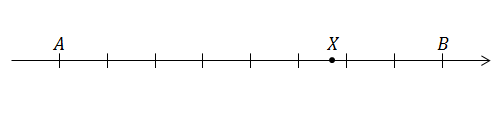If $X=-10.28$ on the real line above, and the distance between each pair of marks is 1, what is the value of $A+B?$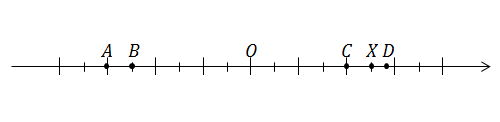Which of the following represents the number that has the same absolute value as $X?$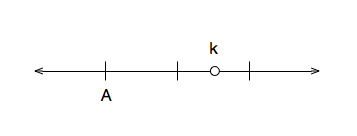In the above number line, if $k = 4.4$, which of the following is a possible value for $A$?

Note: in the image above, each tick mark represents one unit.

×